# Electronics and Communication Engineering - Signals and Systems

Exercise : Signals and Systems - Section 3
1.
Transfer function of a linear system is 200 e-j10ω The system is a
distortionless Attenuator
amplifier with Phase distortion
distortionless Amplifier
attenuator with Phase distortion
Explanation:

Because H(ω) = 200 e-j10ω

And |H(ω)| = 200 with zero phase.

So it cannot be attenuator.

2.
which one is discrete time periodic signal?
sin 3pn
cos 2pn
sin 3pn
all
Explanation:

In Discrete time signal irrational function cannot be periodic, frequency must be rational.

Hence only (c) is periodic.

sin 3pn = sin 3p(n + N) ⇒ for Periodic 3pN = 2Kp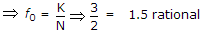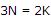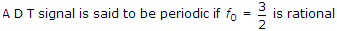.

3.
Frequency domain of a periodic triangular function is a
discrete sinc function
continuous sampling function
discrete sampling function
continuous sampling square function
Explanation:

Because F.T. of a Triangular function is square sampling function.

4.
The z-transform of sequence n x x[n] is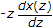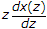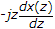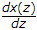Explanation:

It is differentiation property.

5.
The inverse Laplace transform of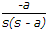eat
-eat
1 - eat
-1 + eat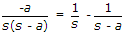.